# Modifying input during OmLoop

Hello! I’m working on a problem, and here it’s a simplified version of it:

Let’s say I have a list (1 2 3 4). I want, starting at the second element (index 1) to add the element at this index with the previous element of the list.
So my desired output, in the example, would be (1 3 6 10). I want to be able to modify the input list at each step, by subbing out the value at index (x) for the value index(x) + index(x-1). Is this possible?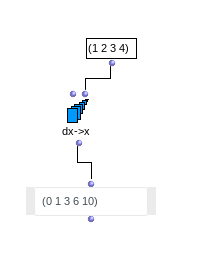Or maybe you have in mind the fibonacci serie???: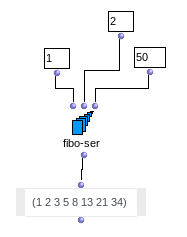Best
K

Hi Karim, sorry I used a simple example, the actual task is more complicated. I’ll describe it here:

I’m working on a program to import a piece in XML, and then create a list of lists, each element being a thruple in the form (duration onset offset), where offset = onset + duration. I already accomplished it.
Here’s the fragment I’m using as a test:

After running it through my program, I got this: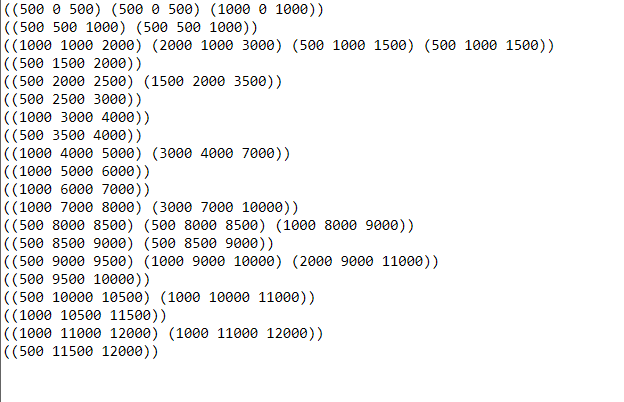Each line represents an onset (which is the MIDDLE number on each thruple).

My goal is to iterate over the indices, starting at the second element (i=1) and ask the following question:

“In the line BEFORE this one, is there any thruple on which the OFFSET (third element) is greater than the CURRENT onset? If YES, I want to append said thruple on the current line”

The thing is, when I move on to the next index, I want my original list to already have the thruple appended, so it can be checked again. For instance: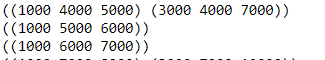Here. if my index is the one poiting to the line ((1000 5000 6000)), I’ll find that the item (3000 4000 7000) has a greater ending (7000) than the current one. So, I’ll append it, making the current line ((1000 5000 6000) (3000 4000 7000))
When I move on to line ((1000 6000 7000)), I’m going to do the check on the previous line, and I want it to SEE the (3000 4000 7000) element, to check the condiditon, and append it, this time to the current line.

My final result (this was done by hand just as to show the desired output) should be this: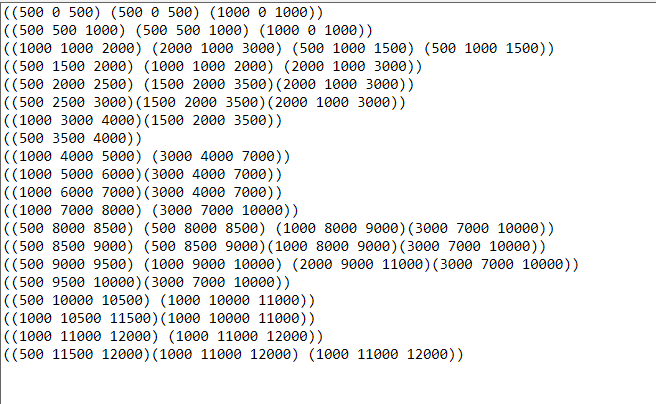Fine,

However it would be easier to me to have your first list as a text based attachment or included in the text. You imagine well i am not going to copy all the list by hand…HAHAHA, of course, sorry.

Here’s the list:

((500 0 500) (500 0 500) (1000 0 1000))
((500 500 1000) (500 500 1000))
((1000 1000 2000) (2000 1000 3000) (500 1000 1500) (500 1000 1500))
((500 1500 2000))
((500 2000 2500) (1500 2000 3500))
((500 2500 3000))
((1000 3000 4000))
((500 3500 4000))
((1000 4000 5000) (3000 4000 7000))
((1000 5000 6000))
((1000 6000 7000))
((1000 7000 8000) (3000 7000 10000))
((500 8000 8500) (500 8000 8500) (1000 8000 9000))
((500 8500 9000) (500 8500 9000))
((500 9000 9500) (1000 9000 10000) (2000 9000 11000))
((500 9500 10000))
((500 10000 10500) (1000 10000 11000))
((1000 10500 11500))
((1000 11000 12000) (1000 11000 12000))
((500 11500 12000))

Ok here it is:

The Patch:
Patch.omp (10.8 KB)

Howerver… either your description of the algorithm is insufficient, Or, the result “by hand” is errounous…Best
K

Hey Karim! Thank you so much for your effort. But there is a mistake in the output:

((1000 1000 2000) (2000 1000 3000) (500 1000 1500) (500 1000 1500))
((500 1500 2000) (2000 1000 3000))

The second line should also have the element (1000 1000 2000), because the ending (2000) is greater then the current onset (1500).

The more actual descritpion of what I was asking would be:

“In the line BEFORE this one, are there any thruple or thrples on which the OFFSET (third element) is greater than the CURRENT onset? If YES, I want to append ALL OF THOSE THRUPLES ON WHICH THE FINAL ELEMENT IS GREATER THAN THE CURRENT ONSET on the current line”

Here you are:
Patch 3.omp (12.4 KB)

But please, next time, make sure your description fits well what you are looking for. The best the description, the better you will find by your own the solutionBest
K

1 Like